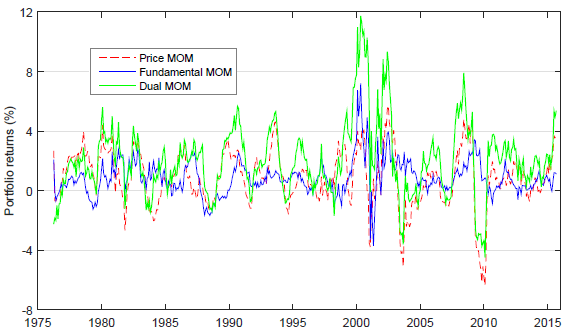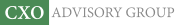Objective research to aid investing decisions

#### Value Investing Strategy (Strategy Overview)

Allocations for September 2023 (Final)
Cash TLT LQD SPY

#### Momentum Investing Strategy (Strategy Overview)

Allocations for September 2023 (Final)
1st ETF 2nd ETF 3rd ETF

# Combining Stock Fundamentals Trend and Price Momentum

| | Posted in: Fundamental Valuation, Momentum Investing

Are trend in stock fundamentals and stock price momentum mutually reinforcing return predictors? In their January 2017 paper entitled “Dual Momentum”, Dashan Huang, Huacheng Zhang and Guofu Zhou combine a measure of fundamentals trend and past return to form a U.S. stock portfolio designed to exploit the powers of both to select outperforming stocks. To construct their measure of fundamentals trend, they each month:

1. For each stock, collect the last eight quarters of seven variables: return on equity; return on assets; earnings per share; accrual-based operating profit to equity; cash-based operating profit to assets; gross profit to assets; and, net payout ratio.
2. For each stock, calculate four moving averages for each fundamental variable over the last 1, 2, 4 and 8 quarters (for a total of 28 moving averages per stock).
3. Across all stocks, relate next-month excess stock return to the most recent 28 fundamentals moving averages via multiple regression to obtain 28 fundamentals trend betas.
4. For each fundamentals beta for each stock, calculate an expected beta as the average of the last 12 monthly betas.
5. For each stock, calculate a fundamentals-implied return (FIR) by applying the 28 expected betas to the most recently available 28 fundamentals moving averages.

They then each month rank stocks into value-weighted fifths (quintiles) based on FIR. Separately, they each month rank the same stocks into value-weighted quintiles based on conventional price momentum (cumulative return from 12 months ago to one month ago). Using quarterly fundamentals and monthly returns for a broad sample of U.S. stocks during January 1973 through September 2015, they find that:

• For fundamentals trend and price momentum separately:
• A hedge portfolio that each month (buys) sells the quintile of stocks with the highest (lowest) FIRs generates average gross monthly return 0.88%, with standard deviation 5.34% and gross monthly Sharpe ratio 0.14.
• A hedge portfolio that each month (buys) sells the quintile of stocks with the highest (lowest) price momentum generates average gross monthly return 0.93%, with standard deviation 6.78% and gross monthly Sharpe ratio 0.16.
• The correlation of monthly returns for these two hedge portfolios is a low 0.14.
• A “dual momentum” portfolio that is each month long (short) stocks that fall in both the top (bottom) FIR quintile and the top (bottom) price momentum quintile generates average gross monthly return 2.16%, with standard deviation 8.34% and gross monthly Sharpe ratio 0.26, twice the Sharpe ratio of the market portfolio. (See the chart below.)
• Gross monthly alphas for this dual momentum portfolio range from 1.37% to 2.50% for various widely used factor models of stock returns.
• The long (short) side of the dual momentum portfolio generates average gross monthly return 1.72% (0.44%), such that a long-only portfolio is attractive on a gross basis.
• The dual momentum portfolio has a high monthly turnover rate of 88%, corresponding to breakeven portfolio reformation frictions of 2.5% of value traded, compared to 2.0% and 1.4% for the fundamentals trend and price momentum hedge portfolios, respectively.
• Dual momentum is stronger for small stocks, but is significant among large stocks.
• Based on the Baker-Wurgler sentiment index, dual momentum average gross monthly return is stronger when investor sentiment is high (2.56%) than when it is low (1.75%).
• Using three or five years of past fundamentals data, rather than two years, produces similar results.
• Using a single regression that includes both fundamentals trend betas and price momentum to rank stocks (instead of sorting separately on fundamentals trend and momentum) reduces dual momentum average gross monthly return by about half.

The following chart, taken from the paper, compares 12-month moving average gross returns for:

• Price MOM – the price momentum hedge portfolio specified above.
• Fundamental MOM – the fundamentals trend hedge portfolio as specified above.
• Dual MOM – the dual momentum portfolio as specified above.

Visual inspection indicates that dual momentum generally outperforms its component strategies, but can bear substantial drawdowns.In summary, evidence indicates that combining information from fundamentals trend and price momentum substantially enhances the power of each separately to select outperforming U.S. stocks.

Cautions regarding findings include:

• Reported returns and Sharpe ratios are gross, not net. Portfolio reformation frictions, which would have been high during the first half of the sample period, and shorting costs would reduce these performance metrics. Shorting of some stocks may have been constrained by lack of shares to borrow.
• The specification of fundamentals trend based on 28 moving averages of seven variables for each stock is very complex, involving considerable data collection/processing. Translation of these variables into FIR via multiple regression adds to complexity. Some simpler specification may suffice to capture a fundamentals trend.
• Investors would further bear fees for delegating complex data collection/processing to an investment/fund manager.
• The complex construction of FIR implies exposures to indirect snooping bias (reliance on prior research that involved snooping) and many opportunities for direct model/data snooping via construction and parameter selection. Reported robustness to fundamentals trend lookback interval somewhat mitigates this concern.

The momentum combination above differs from the intrinsic (absolute or time series) price momentum plus relative price momentum explored in “Melding Momentum, Diversification and Absolute Return” and “A Few Notes on Dual Momentum Investing”.